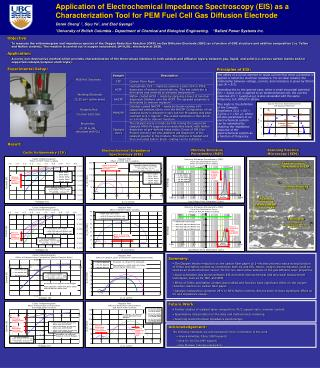DownloadDownload PresentationApplication:

# Application:

Download Presentation## Application:

- - - - - - - - - - - - - - - - - - - - - - - - - - - E N D - - - - - - - - - - - - - - - - - - - - - - - - - - -
##### Presentation Transcript

1. Application of Electrochemical Impedance Spectroscopy (EIS) as a Characterization Tool for PEM Fuel Cell Gas Diffusion Electrode • Derek Cheng1,2, Siyu Ye2, and Elod Gyenge1 • 1University of British Columbia - Department of Chemical and Biological Engineering. 2Ballard Power Systems Inc. MSE Ref. Electrode Working Electrode (2.25 cm2 active area) Graphite Rod Counter Electrode Electrolyte (0.1M H2SO4 saturated with O2) Random carbon fibre matrix in CFP Macro Porosity (filled with epoxy) Effect of Increasing Teflon content of the HCFP Teflon web (increasing hydrophobicity and lower porosity) • Objective: • To measure the voltammogram and impedance spectra of the Oxygen Reduction Reaction (ORR) on Gas Diffusion Electrode (GDE) as a function of GDE structure and additive composition (i.e. Teflon and Nafion content). The reaction is carried out in oxygen saturated 0.1M H2SO4 electrolyte at 293K. • Application: • A novel, non-destructive method which provides characterization of the three-phase interface in both catalyst and diffusion layers, between gas, liquid, and solid (i.e. porous carbon matrix and/or supported catalyst/polymer electrolyte). Experimental Setup: Principles of EIS: The ability of a circuit element to resist current flow when a potential is applied is called the electrical resistance. For an ideal resistor, the relationship between voltage, current, and resistance is given by Ohm’s Law (R = E/I). Extending this to the general case, when a small sinusoidal potential E(t) = E0exp (jt) is applied to an electrochemical cell, the current response I(t) = I0exp(jt-j) is also sinusoidal with the same frequency, but shifted in phase. This leads to the definition of the Complex Impedance Z() = E/I = Z0(Cos  + j Sin ), where kinetics parameters of an electrochemical system can be estimated by studying the impedance response of the electrochemical system as a function of frequency. Rs (electrolyte resistance)  Rct (charge transfer resistance) Result: Mercury Intrusion Porosimetry (MIP) Scanning Electron Microscopy (SEM) Electrochemical Impedance Spectroscopy (EIS) Cyclic Voltammetry (CV) • Summary: • The Oxygen electro-reduction on the carbon fiber paper (a 2-electron process) was a strong function of Teflon and Nafion content as revealed by both CV and EIS. Hence, oxygen electroreduction could be used as an electrochemical ‘sensor’ for the non-destructive analysis of the gas diffusion layer properties. • Good correlation was shown between EIS and other electrochemical and structural measurement techniques, such as CV, MIP, and SEM. • Effect of Teflon and Nafion content was studied and found to have significant effect on the oxygen reduction reaction on carbon fiber paper. • Catalyst composition, between 25% to 50% Nafion content, did not seem to have significant effect on CV and impedance sweep. • Future Work: • Further studies of catalyst layer composition: Pt/C support ratio, ionomer content. • Quantitative interpretation of the data and mathematical modeling. • Scanning electrochemical impedance spectroscopy. • Acknowledgement: • The following individuals are acknowledged for their contribution to this work: • Joanna Kolodziej, P.Eng. (SEM support) • Gina Yin-Chi Chu (MIP support) • Kelly Maclean (Sample preparation)